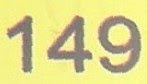## Monday, February 28, 2011

### 149

149 is a prime number.

149 and 151 form a pair of twin primes.

149 has a representation as a sum of two squares: 149 = 72 + 102.

149 is the hypotenuse of a primitive Pythagorean triple: 1492 = 512 + 1402.

149 is the concatenation of the first three positive squares.

149 is the smallest number whose square starts with three identical digits: 1492 = 22201.

149 is the smallest prime that retains the end (unit) digit of its predecessor (139).

149 is the smallest number that is the sum of proper substrings of its reverse: 149 = 1 + 4 + 9 + 94 + 41.149 Medusa is an asteroid discovered in 1875.